# Radiative Heat Transfer - 3 Notes | Study Heat Transfer - Mechanical Engineering

## Document Description: Radiative Heat Transfer - 3 for Mechanical Engineering 2022 is part of Radiation for Heat Transfer preparation. The notes and questions for Radiative Heat Transfer - 3 have been prepared according to the Mechanical Engineering exam syllabus. Information about Radiative Heat Transfer - 3 covers topics like and Radiative Heat Transfer - 3 Example, for Mechanical Engineering 2022 Exam. Find important definitions, questions, notes, meanings, examples, exercises and tests below for Radiative Heat Transfer - 3.

Introduction of Radiative Heat Transfer - 3 in English is available as part of our Heat Transfer for Mechanical Engineering & Radiative Heat Transfer - 3 in Hindi for Heat Transfer course. Download more important topics related with Radiation, notes, lectures and mock test series for Mechanical Engineering Exam by signing up for free. Mechanical Engineering: Radiative Heat Transfer - 3 Notes | Study Heat Transfer - Mechanical Engineering
 1 Crore+ students have signed up on EduRev. Have you?

7.2.2 Wien’s law
Figure 7.4 shows that as the temperature increases the peaks of the curve also increases and it shift towards the shorter wavelength. It can be easily found out that the wavelength corresponding to the peak of the plot (λmax) is inversely proportional to the temperature of the blackbody (Wein’s law) as shown in eq. 7.11.

λmax T = 2898                   (7.11)

Now with the Wien’s law or Wien’s displacement law, it can be understood if we heat a body, initially the emitted radiation does not have any colour. As the temperature rises the λ of the radiation reach the visible spectrum and we can able to see the red colour being height λ (for red colour). Further increase in temperature shows the white colour indicating all the colours in the light.

7.2.3 The Stefan-Boltzmann law for blackbody

Josef Stefan based on experimental facts suggested that the total emissive power of a blackbody is proportional to the fourth power of the absolute temperature. Later, Ludwig Boltzmann derived the same using classical thermodynamics. Thus the eq. 7.12 is known as Stefan-Boltzmann law,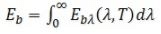Eb = σT4                    (7.12)

where, Eb is the emissive power of a blackbody, T is absolute temperature, and σ (= 5.67 X 10-8W/m2/K4) is the Stefan-Boltzmann constant.

The Stefan-Boltzmann law for the emissive power gives the total energy emitted by a blackbody defined by eq.7.3.

7.2.4 Special characteristic of blackbody radiation

It has been shown that the irradiation field in an isothermal cavity is equal to Eb. Moreover, the irradiation was same for all planes of any orientation within the cavity. It may then be shown that the intensity of the blackbody radiation, Ib, is uniform. Thus, blackbody radiation is defined as,

Eb = πIb                   (7.13)

where,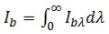is the total intensity of the radiation and  is called the spectral radiation intensity of the blackbody.

7.2.5 Kirchhoff’s law
Consider an enclosure as shown in fig.7.2 and a body is placed inside the enclosure. The radiant heat flux (q) is incident onto the body and allowed to come into temperature equilibrium. The rate of energy absorbed at equilibrium by the body must be equal to the energy emitted.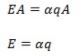(7.14)

where, E is the emissive power of the body, α is absorptivity of the of the body at equilibrium temperature, and A is the area of the body.

Now consider the body is replaced by a blackbody i.e. E → Eb and α = 1, the equation 7.14 becomes

Eb= q                    (7.14)

Dividing eq. 7.14 by eq.7.15,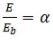(7.16)

At this point we may define emissivity, which is a measure of how good the body is an emitter as compared to blackbody. Thus the emissivity  can be written as the ratio of the emissive power to that of a blackbody,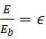(7.17)

On comparing eq.7.16 and eq.7.17, we get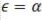(7.18)

Equation 7.18 is the Kirchhoff’s law, which states that the emissivity of a body which is in thermal equilibrium with its surrounding is equal to its absorptivity of the body. It should be noted that the source temperature is equal to the temperature of the irradiated surface. However, in practical purposes it is assumed that emissivity and absorptivity of a system are equal even if it is not in thermal equilibrium with the surrounding. The reason being the absorptivity of most real surfaces is relatively insensitive to temperature and wavelength. This particular assumption leads to the concept of grey body. The emissivity is considered to be independent of the wavelength of radiation for grey body.

7.3 Grey body
If grey body is defined as a substance whose monochromatic emissivity and absorptivity are independent of wavelength. A comparative study of grey body and
blackbody is shown in the table 7.2.

Table-7.2: Comparison of grey and blackbody

 Blackbody Grey body Ideal body Ideal body Emissivity (∈) is independent of wavelength Emissivity (∈) is independent of wavelength Absorptivity (α) is independent of wavelength Absorptivity (α) is independent of wavelength ε = 1 ε < 1 α = 1 α < 1

Illustration 7.1
The surface of a blackbody is at 500 K temperature. Obtain the total emissive power, the wavelength of the maximum monochromatic emissive power.

Solution 7.1

Using eq. 7.12, the total emissive power can be calculated,

Eb = σT4

where, σ (= 5.67 X 10-8 W/m2/K4) is the Stefan-Boltzmann constant. Thus at 500 K,

Eb = (5.67 X 10-8)(50004) W/m2

Eb = 354.75 W/m2

The wavelength of the maximum monochromatic emissive power can be obtained from the Wien’s law (eq. 7.11),

λmaxT = 2898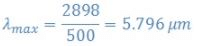The document Radiative Heat Transfer - 3 Notes | Study Heat Transfer - Mechanical Engineering is a part of the Mechanical Engineering Course Heat Transfer.
All you need of Mechanical Engineering at this link: Mechanical Engineering

## Heat Transfer

58 videos|70 docs|85 tests
 Use Code STAYHOME200 and get INR 200 additional OFF

## Heat Transfer

58 videos|70 docs|85 tests

Track your progress, build streaks, highlight & save important lessons and more!

,

,

,

,

,

,

,

,

,

,

,

,

,

,

,

,

,

,

,

,

,

;